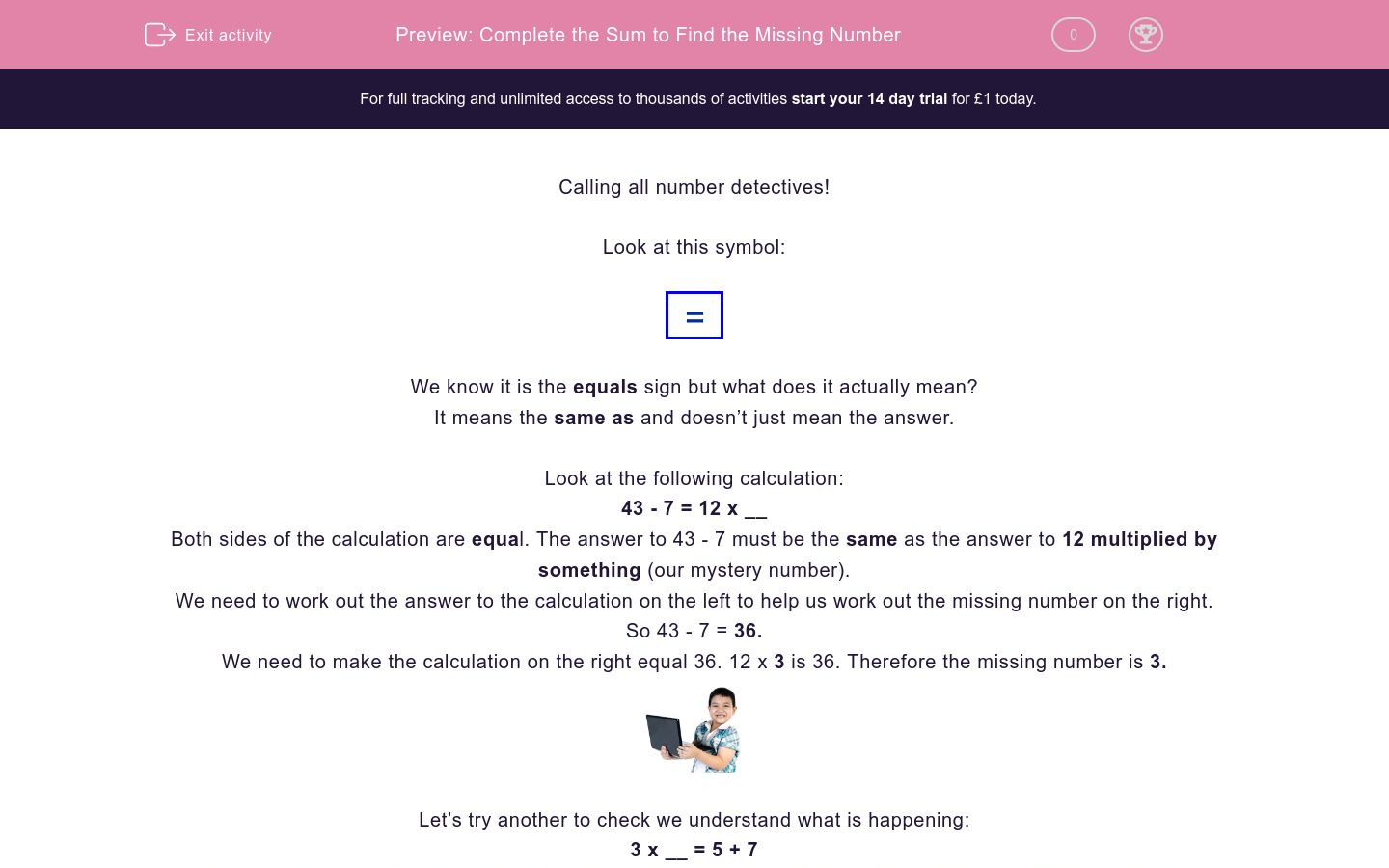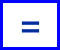# Complete the Sum to Find the Missing Number

In this worksheet, students will complete a calculation by adding in the missing number so that both sides are equal. It will strengthen their number skills.Key stage:  KS 2

Curriculum topic:   Verbal Reasoning

Curriculum subtopic:   Complete the Calculation

Difficulty level:### QUESTION 1 of 10

Calling all number detectives!

Look at this symbol:We know it is the equals sign but what does it actually mean?

It means the same as and doesn’t just mean the answer.

Look at the following calculation:

43 - 7 = 12 x __

Both sides of the calculation are equal. The answer to 43 - 7 must be the same as the answer to 12 multiplied by something (our mystery number).

We need to work out the answer to the calculation on the left to help us work out the missing number on the right.

So 43 - 7 = 36.

We need to make the calculation on the right equal 36. 12 x 3 is 36. Therefore the missing number is 3.Let’s try another to check we understand what is happening:

3 x __ = 5 + 7

Here we must work out the right side of the calculation to help us work out the missing number on the left.

So 5 + 7 = 12.

3 x ? = 12

The missing number is 4 as 3 x 4 = 12.

3 x 4 = 5 + 7

Remember that equals means the same as and the answers on either side of the equals sign must be the same.

In this activity, you will be completing sums by finding the missing number.

Good luck!

---- OR ----